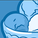New to Qlik Sense

If you’re new to Qlik Sense, start with this Discussion Board and get up-to-speed quickly.

Announcements
QlikWorld 2022, LIVE in Denver CO., May 16-19, 2022. REGISTER NOW TO RECEIVE EARLY BIRD PRICING
cancel
Showing results for
Did you mean:Creator

Count with Average (Aggr function)

Hi guys,

I want to use count and avg together but can't get it to work.

I have to count the studies when all these conditions are met.

Can anyone help?

count( aggr
if([Dimension1]='abc' and [Dimension2]='Closed' and Yearstart([Datefield]=yearstart(today()))
and avg([No. of Days])<=7,1,0) , StudyNumber))

I believe I'll have to use Aggr but don't know how.

Thanks so much!

Labels (5)

• Set Analysis As A Dimension

1 Solution

Accepted SolutionsMVP

@SkitzN27  try below. Make sure that the Date field is in proper date format

=sum({<[Dimension1]={'abc'},[Dimension2]={'Closed'},[Datefield]={"\$(=date(yearstart(today())))"}>} aggr(
if(avg({<[Dimension1]={'abc'},[Dimension2]={'Closed'},[Datefield]={"\$(=date(yearstart(today())))"}>}[No. of Days])<=7,1,0) , StudyNumber))

4 RepliesSpecialist

Try this:

Count(if(aggr(avg({\$<[Dimension1]={'abc'},[Dimension2]={'Closed'},[Datefield]={">=\$(=YearStart(Today()))<=\$(=Today())"}>} [No. of Days])<=7, StudyNumber))

You may want to add a distinct if there can be multiple records for a StudyNumber.Creator
Author

Hey, it doesn't work.Specialist

Sorry, I don't have your exact fields to test it, so the syntax was a little off:

Try this:

Count(if(aggr(avg({\$<[Dimension1]={'abc'},[Dimension2]={'Closed'},[Datefield]={">=\$(=YearStart(Today()))<=\$(=Today())"}>} [No. of Days]), StudyNumber)<=7, StudyNumber))MVP

@SkitzN27  try below. Make sure that the Date field is in proper date format

=sum({<[Dimension1]={'abc'},[Dimension2]={'Closed'},[Datefield]={"\$(=date(yearstart(today())))"}>} aggr(
if(avg({<[Dimension1]={'abc'},[Dimension2]={'Closed'},[Datefield]={"\$(=date(yearstart(today())))"}>}[No. of Days])<=7,1,0) , StudyNumber))Tags
Community Browser